## 929 Reputation

4 years, 103 days
changsha, China

## On the bondage number of a graph....

Maple 2023
• A set D of vertices in a graph G is a dominating set if each vertex of G that is not in D is adjacent to at least one vertex of D.
• The minimum cardinality among all dominating sets in G is called the domination number of G and denoted σ(G).
• We define the bondage number b(G) of a graph G to be the cardinality of a smallest set E of edges for which σ(GE)>σ(G).

In the previous post, Carl Love provided a code for calculating domination number, so we could use it to calculate bondage number. I wrote a piece of code using a relatively basic approach. I feel like there is room for improvement.  I still can't determine the bondage number of the following graph so far.

 > restart: with(GraphTheory): with(Iterator): #Author: Carl Love , the Glorious 1st of June, 2023 # Domination_number:= (G::Graph)-> local V:= {\$nops(op(3,G))}, A:= op(4,G) union~ {}~(V), s, v, U:= table([{}= {}]), Us;     in V do         for s in {indices}(U, 'nolist') do             Us:= U[s]; U[s]:= evaln(U[s]);             for v in V minus s do                 if (U[s union {v}]:= Us union A[v]) = V then return nops({s[], v}) fi             od         od     od :
 > Bondage_number:=proc(g::Graph) local dom,vn,edge_ofg,t,M,removeedge,H,i,hasNext, getNext,result; dom:=Domination_number(g); vn:=nops(op(3,g)); edge_ofg:=convert(Edges(g),list); result:="Null"; for t from 1 to vn do     M := Combination(vn, t);     hasNext, getNext := ModuleIterator(M);       while hasNext() do         removeedge:= {seq(edge_ofg[i], i in getNext()+~1)};         H := DeleteEdge(g, removeedge,inplace = false);         if Domination_number(H)> dom then                 result:=nops(removeedge);          break t;         end if;         end do; end do: return result; end proc:
 > ed:={{1, 2}, {1, 3}, {1, 4}, {1, 5}, {1, 7}, {1, 9}, {1, 11}, {2, 3}, {2, 5},  {2, 6}, {2, 7}, {2, 12}, {2, 14}, {3, 4}, {3, 7}, {3, 8}, {3, 9}, {3, 16}, {4, 5},  {4, 9}, {4, 10}, {4, 11}, {4, 17}, {5, 6}, {5, 11}, {5, 12}, {5, 19}, {6, 7}, {6, 12},{6, 13}, {6, 14}, {6, 21}, {7, 8}, {7, 14}, {7, 15}, {8, 9}, {8, 14}, {8, 15}, {8, 16}, {8, 22}, {9, 10}, {9, 16}, {9, 17}, {10, 11}, {10, 17}, {10, 18}, {10, 19}, {10, 23}, {11, 12},{11, 18}, {11, 19}, {12, 13}, {12, 19}, {12, 20}, {13, 14}, {13, 19}, {13, 20}, {13, 21}, {13, 24},{14, 15}, {14, 21}, {15, 16}, {15, 21}, {15, 22}, {15, 24}, {16, 17}, {16, 22}, {16, 23}, {17, 18}, {17, 22}, {17, 23}, {18, 19}, {18, 20}, {18, 23}, {18, 24}, {19, 20}, {20, 21}, {20, 23}, {20, 24},{21, 22}, {21, 24}, {22, 23}, {22, 24}, {23, 24}}: g:=Graph(ed);(1)
 > Domination_number(g)(2)
 > Bondage_number(g);#Long time..

Bondage_number.mw

For arbitrarily given graph, it has been proved that determining its bondage number is NP-hard.

I always have a feeling that when removing subsets of edges, the symmetry of these edge subsets can be utilized. Above, we blindly traverse k-subsets of edges. If the graph is symmetric enough, perhaps we can reduce the amount of traversal. However, I have been struggling to understand how to express the symmetry of these edge subsets. Recently, I raised a similar question.

## How can I calculate the domination numbe...

Maple 2023

In graph theory, a dominating set for a graph G is a subset D of its vertices, such that any vertex of G is either in D, or has a neighbor in D. The domination number γ(G) is the number of vertices in a smallest dominating set for G. The domination number is a well-known parameter in graph theory. But I am unable to find a built-in function in Maple to calculate the domination number of a graph. Did I miss something?

For example, I would like to calculate the domination number of the following graph.

ed:={{1, 2}, {1, 3}, {1, 4}, {1, 5}, {1, 7}, {1, 9},
{1, 11}, {2, 3}, {2, 5}, {2, 6}, {2, 7}, {2, 12}, {2, 14},
{3, 4}, {3, 7}, {3, 8}, {3, 9}, {3, 16}, {4, 5}, {4, 9},
{4, 10}, {4, 11}, {4, 17}, {5, 6}, {5, 11}, {5, 12}, {5, 19},
{6, 7}, {6, 12}, {6, 13}, {6, 14}, {6, 21}, {7, 8}, {7, 14},
{7, 15}, {8, 9}, {8, 14}, {8, 15}, {8, 16}, {8, 22}, {9, 10},
{9, 16}, {9, 17}, {10, 11}, {10, 17}, {10, 18}, {10, 19},
{10, 23}, {11, 12}, {11, 18}, {11, 19}, {12, 13}, {12, 19},
{12, 20}, {13, 14}, {13, 19}, {13, 20}, {13, 21}, {13, 24},
{14, 15}, {14, 21}, {15, 16}, {15, 21}, {15, 22}, {15, 24},
{16, 17}, {16, 22}, {16, 23}, {17, 18}, {17, 22}, {17, 23},
{18, 19}, {18, 20}, {18, 23}, {18, 24}, {19, 20}, {20, 21},
{20, 23}, {20, 24}, {21, 22}, {21, 24}, {22, 23}, {22, 24}, {23, 24}};
g:=Graph(ed);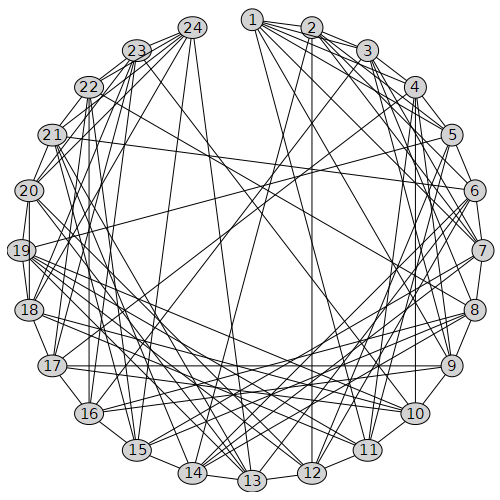## The LaTeX commands seem to be too stable...

Maple 2023

I find that no matter how I modify the style in the Maple command, the LaTeX output seems to be always fixed. This is a bit frustrating.

G:=Graph(6,{{1,2},{1,4},{4,5},{2,5},{2,3},{3,6},{5,6}});
DrawGraph(G);
vp:=[[0,0],[0.5,0],[1,0],[0,0.5],[0.5,0.5],[1,0.5]]:
SetVertexPositions(G,vp):
DrawGraph(G);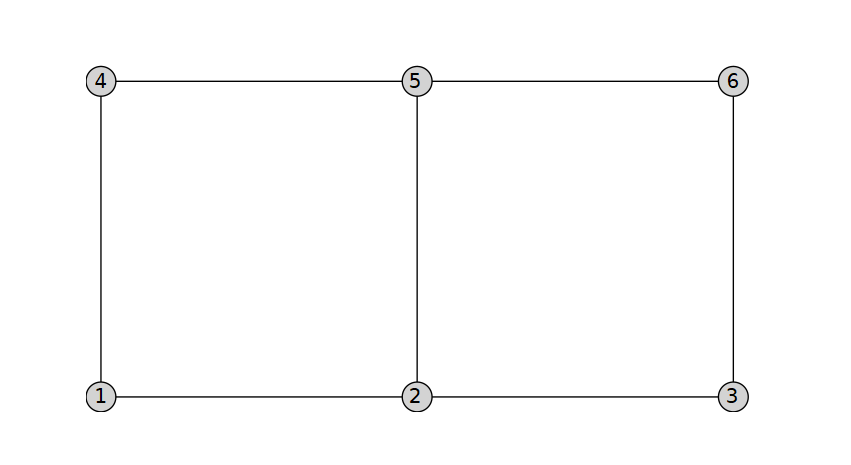Latex(G, terminal, 100, 100)


\documentclass{amsart}
\begin{document}
\begin{picture}(100,100)
\qbezier(12.40,87.60)(12.40,50.00)(12.40,12.40)
\qbezier(12.40,87.60)(31.20,50.00)(50.00,12.40)
\qbezier(12.40,12.40)(31.20,50.00)(50.00,87.60)
\qbezier(12.40,12.40)(50.00,50.00)(87.60,87.60)
\qbezier(50.00,87.60)(68.80,50.00)(87.60,12.40)
\qbezier(50.00,12.40)(68.80,50.00)(87.60,87.60)
\qbezier(87.60,87.60)(87.60,50.00)(87.60,12.40)
\put(12.400000,87.600000){\circle*{6}}
\put(10.194,96.943){\makebox(0,0){1}}
\put(12.400000,12.400000){\circle*{6}}
\put(8.726,3.531){\makebox(0,0){2}}
\put(50.000000,87.600000){\circle*{6}}
\put(50.000,97.200){\makebox(0,0){3}}
\put(50.000000,12.400000){\circle*{6}}
\put(50.000,2.800){\makebox(0,0){4}}
\put(87.600000,87.600000){\circle*{6}}
\put(91.274,96.469){\makebox(0,0){5}}
\put(87.600000,12.400000){\circle*{6}}
\put(89.806,3.057){\makebox(0,0){6}}
\end{picture}
\end{document}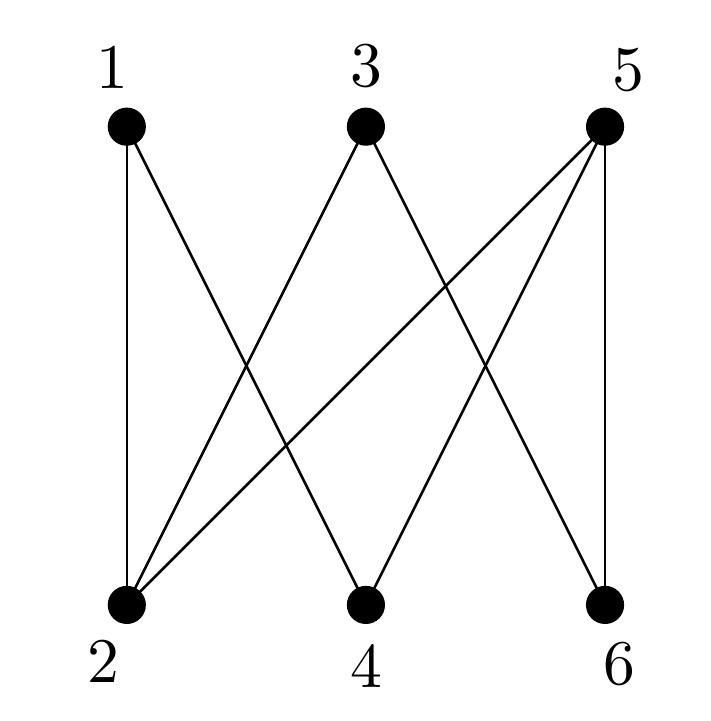DrawGraph(G,
vertexcolor="Gold",edgethickness=3], font=["Courier",10],size=[180,180])Even when I change their colors (any other thing), they always go their own way. I don't know if there is a setting that can make the LaTeX output more flexible and in line with our expectations after adjustment.

## How can we find a shortest closed walk p...

Maple 2023

A Hamiltonian walk on a connected graph is a closed walk of minimal length which visits every vertex of a graph (and may visit vertices and edges multiple times). (See https://mathworld.wolfram.com/HamiltonianWalk.html)

Please note that there is a distinction between a Hamiltonian walk and a Hamiltonian cycle. Any graph can have a Hamiltonian walk, but not necessarily a Hamiltonian cycle.  The Hamiltonian number h(n) of a connected G is the length of a Hamiltonian walk.

For example, let's consider the graph FriendshipGraph(2).

g:=GraphTheory[SpecialGraphs]:-FriendshipGraph(2);
DrawGraph(g)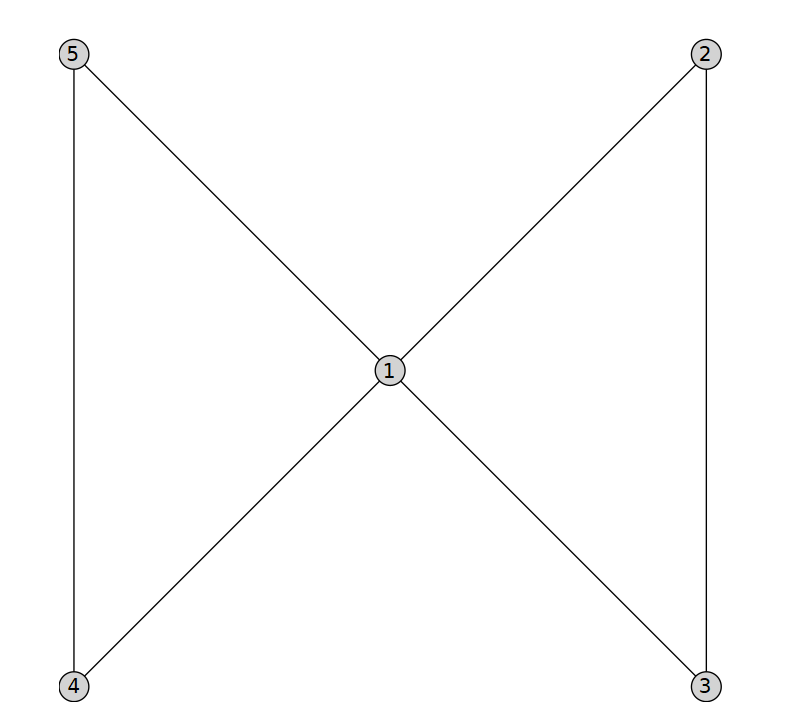Then its Hamiltonian number is 6.  A Hamiltonian walk is as shown in the following picture.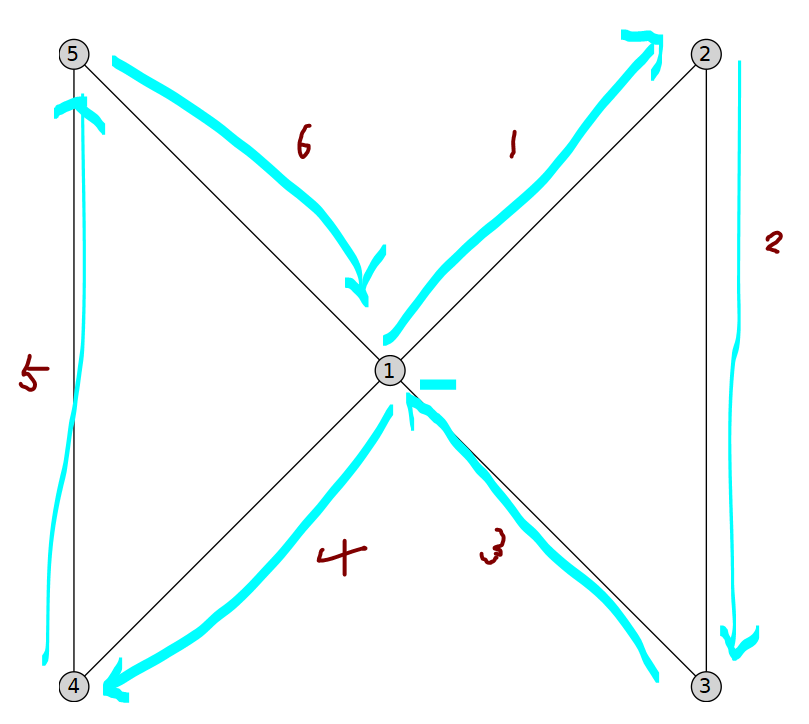PS: After being reminded by others, I learned that a method in the following post seems to work, but I don't understand why it works for now.

it's possible to formulate this as a Travelling Salesman Problem by augmenting the sparse graph into a complete graph by adding edges. These new edges represent shortest paths between any two vertices in the original graph and have weight equal to the path length.

It seems that the correctness of this transformation requires rigorous proof.

## Why does the figure generated from the t...

Maple 2023

I followed the code on the website https://de.maplesoft.com/support/help/maple/view.aspx?path=updates/Maple18/GraphTheory to convert a graph to LaTeX code. However, after compiling with pdflatex, I found that some edges of the graph are jagged.

restart:
with(GraphTheory):
with(SpecialGraphs):
S:=SoccerBallGraph():
Latex(S,FileTools:-JoinPath([currentdir(), "soccer.tex"]),300,300,true)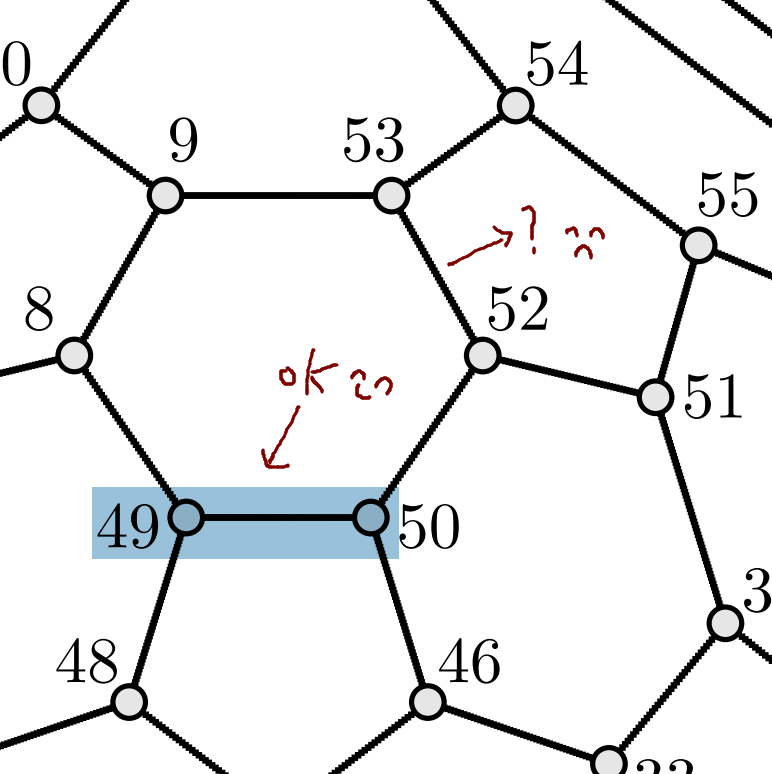soccer.pdf

I suspect it's because of the converted LaTeX code.

PS: The PDF conversion issue from last time still remains unsolved in Maple 2023; see

 1 2 3 4 5 6 7Last Page 1 of 24
﻿